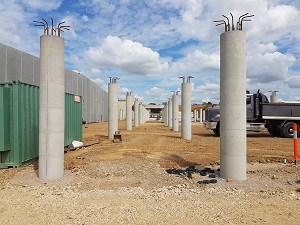# Round Column Calculator

##Round Column Calculation

meter
cm
meter
cm

#### Total Volume of Round Column

2.85 m3 | 100.68 ft3

Sr. Material Quantity
1Cement 23 Bags
2Sand 1.84 Ton
3Aggregate 3.20 Ton

## Round Column Calculation

$=\frac{\mathrm{Diameter}}{2}$

$=\frac{\mathrm{1.10}}{2}$

$=\mathrm{0.55}$

#### Volume

$={\mathrm{\pi r}}^{2}h×\mathrm{No. of Columns}$

$=3.14×\mathrm{0.55}×\mathrm{0.55}×3×1$

$=2.85 Cubic Meter$

$=100.68 Cubic Feet$

#### Dry Volume

$=\mathrm{Volume \left(Cubic Meter\right)}×1.524$

$=\mathrm{2.85}×1.524$

$=\mathrm{4.34}$

#### Cement Volume

$=\frac{\mathrm{Dry Volume}×\mathrm{Cement}}{\mathrm{Sum of Ratio}}$

$=\frac{\mathrm{4.34}×1}{\mathrm{5.5}}$

$=\mathrm{0.79}$

#### No. of Cement Bags

$=\frac{\mathrm{Cement Volume}}{0.035}$

$=\frac{\mathrm{0.79}}{0.035}$

$=\mathrm{23 Bags}$
Note: 1 Bag of cement = 0.035 m3.
1 Cement bag contains = 50 kg cement

#### Sand Volume

$=\frac{\mathrm{Dry Volume}×\mathrm{Sand}}{\mathrm{Sum of Ratio}}$

$=\frac{\mathrm{4.34}×\mathrm{1.5}}{\mathrm{5.5}}$

$=\mathrm{1.18}$

#### Sand in Ton

$=\frac{\mathrm{Sand Volume}×1550}{1000}$

$=\frac{\mathrm{1.18}×1550}{1000}$

$=\mathrm{1.84 Tons}$
Note: By considering dry loose bulk density of sand = 1550 kg/m3.
1000 kg = 1 Ton

#### Aggregate Volume

$=\frac{\mathrm{Dry Volume}×\mathrm{Aggregate}}{\mathrm{Sum of Ratio}}$

$=\frac{\mathrm{4.34}×3}{\mathrm{5.5}}$

$=\mathrm{2.37}$

#### Aggregate in Ton

$=\frac{\mathrm{Aggregate Volume}×1350}{1000}$

$=\frac{\mathrm{2.37}×1350}{1000}$

$=\mathrm{3.20 Tons}$
Note: By considering dry loose bulk density of aggregate = 1350 kg/m3.
1000 kg = 1 Ton

## What is circular column calculation?

Circular columns are symmetric about any centroidal axis. A reinforced circular concrete column is a structural member designed to carry compressive loads, composed of concrete with an embedded steel frame to provide reinforcement.

Spiral columns are cylindrical columns with a continuous helical bar wrapping around the column. The spiral acts to provide support in the transverse direction and prevent the column from barreling. The amount of reinforcement is required to provide additional load-carrying capacity greater than or equal to that attributed from the shell as to compensate for the strength lost when the shell spalls off.##### Circular Column Calculation
$Radius =\frac{\mathrm{Diameter}}{2}$

$Volume of round column ={\mathrm{\pi r}}^{2}h$

$Dry Volume =\mathrm{Volume \left(Cubic Meter\right)}×1.524$

$Cement Volume =\frac{\mathrm{Dry Volume}×\mathrm{Cement}}{\mathrm{Sum of Ratio}}$

$No. of Cement Bags =\frac{\mathrm{Cement Volume}}{0.035}$

$Sand Volume =\frac{\mathrm{Dry Volume}×\mathrm{Sand}}{\mathrm{Sum of Ratio}}$

$Sand in Ton=\frac{\mathrm{Sand Volume}×1550}{1000}$

$Aggregate Volume =\frac{\mathrm{Dry Volume}×\mathrm{Aggregate}}{\mathrm{Sum of Ratio}}$

$Aggregate in Ton=\frac{\mathrm{Aggregate Volume}×1350}{1000}$
Where,
• 1.524 is dry volume for the concrete
• 0.035 is volume of one cement bag.
• 1550 is density volume conversion sand m3 to kg.
• 1350 is density volume conversion aggregate m3 to kg.## What are the importance circular column?

Circular columns occupy lesser area when compared to the square columns hence it is preferred for pier and columns where in there is traffic expected,Strength of circular column is 1.05 times strength of square column.they are mostly used in piling and elevation of the buildings. Why we use it in elevation? In order to avoid edges, we use this type of columns. they are also provided in sit out areas, auditoriums or fire assembly zones, where you have enough space for them not to hinder any movement of people or look bad with flat surfaces You can find circular columns as pillars of Bridges because there you don’t need to flush them to anything. Also circular looks aesthetic there.